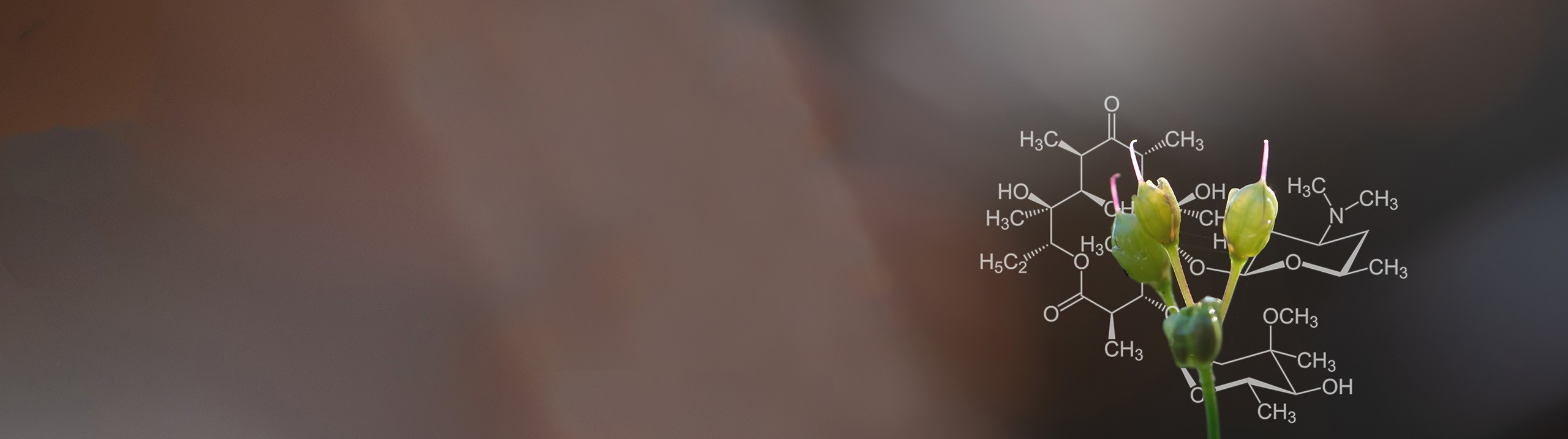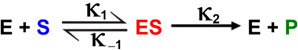Start typing, then use the up and down arrows to select an option from the list.# Biochemistry

Learn the toughest concepts covered in Biochemistry with step-by-step video tutorials and practice problems by world-class tutors

6. Enzymes and Enzyme Kinetics

# Rate Constant Units

1

#### concept

Rate Constant Units3m
Play a video:
in this video, we're going to talk about the units of the rate constant. So as we already know from our previous lesson videos, the units of the rate constant K will actually differ depending on the overall reaction order. And so what we're saying here is that the units of the rate constant K will be different for zero order, first order and second order reactions. And so down below, we're gonna focus specifically on the units of the rate constant, which are as follows, uh, in the following table. And so notice in this table down below. In the first column here, what we have is the overall reaction order. And in the second column, what we have is the units of the rate constant K. And so, for zero order reactions, the units of the rate constant K turned out to be molar ity per second. And, of course, mole arat E per second is the same thing as saying molar ity times, inverse seconds. And so this is the same exact thing as this now for first order reactions. The units of the rate constant K turned out to be one over seconds, and so of course, this is the same thing as saying just inverse seconds. And so this is the same thing as this. And then, of course, last but not least, for second order reactions, the units of the rate constant K turned out to be one over molar ity, times seconds. And so this, of course, turns out to be inverse mole arat e times in verse seconds. And so again, this is the same thing as this. And so one thing to note about all of these units that they share in common is that they all contain inverse seconds as part of the units in one way or another. And so notice that for second order reactions, the units contain inverse seconds for first order reactions. The rate constant units contain inverse seconds and for zero order reaction to the units also contain inverse seconds. And so that's definitely helpful to help us memorize these units a little bit better Now, another thing to take into account is that the some of the units exponents will actually indicate the overall reaction order. And so notice for zero order reactions. If we some all of the unit exponents, it would be one on the polarity minus one and one minus one is, of course, zero. Which tells us that this unit is specifically for zero order reactions. Now, for first order reaction to notice that the units three exponents here are just negative one or just one, for that matter. Which tells us that this is the units for first order reactions. And then, of course, last but not least, for second order reactions. If we some the units here, negative one minus one is, of course, negative, too. So that reminds us that this is for second order reactions. And so, keeping that in mind, we'll be able to apply these concepts here in our example problems. So I'll see you guys there.
2

#### example

Rate Constant Units Example 12m
Play a video: